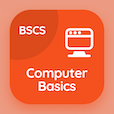SAT Online Courses

SAT Physics Quizzes

SAT Physics Quiz PDF - Complete

# Acceleration of Free Fall Quiz Questions Online p. 19

Learn Acceleration of Free Fall quiz questions and answers, acceleration of free fall MCQ with answers PDF 19 to learn SAT Physics online course. Kinematics trivia questions, Acceleration of Free Fall Multiple Choice Questions (MCQ) for online college degrees. "Acceleration of Free Fall Quiz" PDF Book: energy in physics, mass and weight, power in physics, temperature and measuring instruments, acceleration of free fall test prep for free career test.

"With the speed of the object, the air resistance" MCQ PDF: increases, decreases, remains constant, and becomes zero for job placement test. Study kinematics questions and answers to improve problem solving skills for online SAT practice test.

## Quiz on Acceleration of Free Fall MCQs

MCQ: With the speed of the object, the air resistance

decreases
increases
remains constant
becomes zero

MCQ: The thermal energy that is being transferred from one region of higher temperature to a region of lower temperature is called

light
heat
chemical energy
electricity

MCQ: The SI unit of power is

Joule
Newton
watt
pascal

MCQ: The more mass an object has, the gravitational force acting on it is

smaller
equal
zero
greater

MCQ: The moving objects has

heat energy
kinetic energy
chemical energy
magnetic energy

### More Quizzes from SAT Physics Course